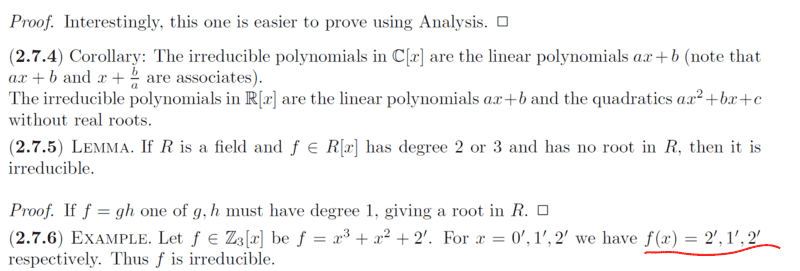# Show that ##f(x)=2^{'},1^{'},2{'}## in the irreducible Polynomial

Gold Member
Homework Statement:
See attached
Relevant Equations:
Ring TheoryMy interest is on the highlighted; my understanding is that,

let

##f(x)=x^3+x^2+2^{'}##

then

##f(1^{'})=1{'}+1{'}+2^{'}=4^{'} ##

we know that in ##\mathbb{z_3} ## that ##\dfrac{4}{3}=1^{'}##

##f(2^{'})=8^{'}+4^{'}+2^{'}=14{'} ##

we know that in ##\dfrac{14^{'}}{3}=1^{'}##...

I hope that is the correct reasoning for the highlighted part indicated in red.

Mentor
2022 Award
Homework Statement:: See attached
Relevant Equations:: Ring Theory

View attachment 323813

My interest is on the highlighted; my understanding is that,

let

##f(x)=x^3+x^2+2^{'}##

then
##f(0')=2'.##
##f(1^{'})=1{'}+1{'}+2^{'}=4^{'} ##

we know that in ##\mathbb{z_3} ## that ##\dfrac{4}{3}=1^{'}##
No fractions, please. We have ##4'=1' ## in ##\mathbb{Z}_3##.
##f(2^{'})=8^{'}+4^{'}+2^{'}=14{'} ##

we know that in ##\dfrac{14^{'}}{3}=1^{'}##...

Same here, only that ##14'=2'##

I hope that is the correct reasoning for the highlighted part indicated in red.
These three equations show that the polynomial has no zero. If it was reducible, say ##f(x)=g(x)\cdot h(x)##, then either ##g(x)## or ##h(x)## had to be linear, say ##g(x)=x-c'.## But this means ##g(c')=0## and ##f(c')=g(c')\cdot h(c')=0## which we excluded.

•chwala
Gold Member
##f(0')=2'.##

No fractions, please. We have ##4'=1' ## in ##\mathbb{Z}_3##.

Same here, only that ##14'=2'##

These three equations show that the polynomial has no zero. If it was reducible, say ##f(x)=g(x)\cdot h(x)##, then either ##g(x)## or ##h(x)## had to be linear, say ##g(x)=x-c'.## But this means ##g(c')=0## and ##f(c')=g(c')\cdot h(c')=0## which we excluded.
Noted @fresh_42 ...on the fraction bit. Cheers...

•fresh_42
Gold Member
##f(0')=2'.##

No fractions, please. We have ##4'=1' ## in ##\mathbb{Z}_3##.

Same here, only that ##14'=2'##

These three equations show that the polynomial has no zero. If it was reducible, say ##f(x)=g(x)\cdot h(x)##, then either ##g(x)## or ##h(x)## had to be linear, say ##g(x)=x-c'.## But this means ##g(c')=0## and ##f(c')=g(c')\cdot h(c')=0## which we excluded.
If a given polynomial has ##f(x)=0##, then it would imply existence of zero divisors- hence no integral domain...correct?

Mentor
2022 Award
If a given polynomial, say with two variables, ##x## and ##y## has ##f(x)=0##,...
What do you mean? You say two variables but write only one.
then it would imply existence of zero divisors- hence no integral domain...correct?

like in this case, we are having ##x^{s}## and ##2## as our ##y##...
The definition of an integral domain is simple. It means that no non-zero elements can be multiplied to zero.
In formulas: ##(a\cdot b= 0 \Longrightarrow a=0 \text{ or }b=0) \Longleftrightarrow (a\neq 0 \text{ and }b\neq 0 \Longrightarrow a\cdot b\neq 0).##

E.g., the 12 hour marks on a clock's face ##\{0,1,2,3,\ldots,10,11\}## has zero divisors: ##3\cdot 4 = 12 = 0## or ##2\cdot 6 = 0.## The light switch ##\{0,1\}## has no zero-divisors. Although we have ##1+1=0## we do not have ##1\cdot 1=0,## and ##2## does not exist (or equals ##0##, depending on how we define it).

##\mathbb{Z}_n## is an integral domain if and only if ##n## is prime. In this case, it is even a field.

•chwala
Homework Helper
2022 Award
Note that in $\mathbb{Z}_3$, $2' = -1'$ and $(-1')^n$ is $1'$ if $n$ is even and $-1'$ if $n$ is odd. Therefore $(2')^3 + (2')^2 = 0'$.

•chwala
•